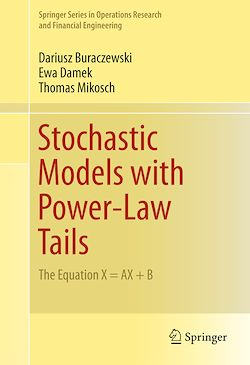# Stochastic Models with Power-Law Tails

-English
320 Pages

Description

In this monograph the authors give a systematic approach to the probabilistic properties of the fixed point equation X=AX+B. A probabilistic study of the stochastic recurrence equation X_t=A_tX_{t-1}+B_t for real- and matrix-valued random variables A_t, where (A_t,B_t) constitute an iid sequence, is provided. The classical theory for these equations, including the existence and uniqueness of a stationary solution, the tail behavior with special emphasis on power law behavior, moments and support, is presented. The authors collect recent asymptotic results on extremes, point processes, partial sums (central limit theory with special emphasis on infinite variance stable limit theory), large deviations, in the univariate and multivariate cases, and they further touch on the related topics of smoothing transforms, regularly varying sequences and random iterative systems.

The text gives an introduction to the Kesten-Goldie theory for stochastic recurrence equations of the type X_t=A_tX_{t-1}+B_t. It provides the classical results of Kesten, Goldie, Guivarc'h, and others, and gives an overview of recent results on the topic. It presents the state-of-the-art results in the field of affine stochastic recurrence equations and shows relations with non-affine recursions and multivariate regular variation.

Subjects

##### Theory

Informations